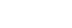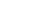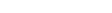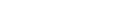xColossally abundant numberEncyclopedia
In mathematics
Mathematics
Mathematics is the study of quantity, space, structure, and change. Mathematicians seek out patterns and formulate new conjectures. Mathematicians resolve the truth or falsity of conjectures by mathematical proofs, which are arguments sufficient to convince other mathematicians of their validity...

, a colossally abundant number (sometimes abbreviated as CA) is a natural number
Natural number
In mathematics, the natural numbers are the ordinary whole numbers used for counting and ordering . These purposes are related to the linguistic notions of cardinal and ordinal numbers, respectively...

that, in some rigorous sense, has a lot of divisor
Divisor
In mathematics, a divisor of an integer n, also called a factor of n, is an integer which divides n without leaving a remainder.-Explanation:...

s. Formally, a number n is colossally abundant if and only if
If and only if
In logic and related fields such as mathematics and philosophy, if and only if is a biconditional logical connective between statements....

there is an ε > 0 such that for all k > 1,where σ denotes the sum-of-divisors function
Divisor function
In mathematics, and specifically in number theory, a divisor function is an arithmetical function related to the divisors of an integer. When referred to as the divisor function, it counts the number of divisors of an integer. It appears in a number of remarkable identities, including relationships...

. The first few colossally abundant numbers are 2, 6, 12
12 (number)
12 is the natural number following 11 and preceding 13.The word "twelve" is the largest number with a single-morpheme name in English. Etymology suggests that "twelve" arises from the Germanic compound twalif "two-leftover", so a literal translation would yield "two remaining [after having ten...

, 60
60 (number)
60 is the natural number following 59 and preceding 61. Being three times twenty, 60 is called "three score" in some older literature.-In mathematics:...

, 120
120 (number)
120 is the natural number following 119 and preceding 121. 120 was known as "the great hundred", especially prior to the year 1700, from the Teutonic Hundert which equalled 120. The number 100, now known commonly as "one hundred" was then known as "the small hundred". It is also known as...

, 360, 2520, 5040, ... ; all colossally abundant numbers are also superabundant numbers, but the converse is not true.

## History

Colossally abundant numbers were first studied by Ramanujan
Srinivasa Ramanujan
Srīnivāsa Aiyangār Rāmānujan FRS, better known as Srinivasa Iyengar Ramanujan was a Indian mathematician and autodidact who, with almost no formal training in pure mathematics, made extraordinary contributions to mathematical analysis, number theory, infinite series and continued fractions...

and his findings were intended to be included in his 1915 paper on highly composite number
Highly composite number
A highly composite number is a positive integer with more divisors than any positive integer smaller than itself.The initial or smallest twenty-one highly composite numbers are listed in the table at right....

s. Unfortunately, the publisher of the journal to which Ramanujan submitted his work, the London Mathematical Society
London Mathematical Society
-See also:* American Mathematical Society* Edinburgh Mathematical Society* European Mathematical Society* List of Mathematical Societies* Council for the Mathematical Sciences* BCS-FACS Specialist Group-External links:* * *...

, was in financial difficulties at the time and Ramanujan agreed to remove aspects of the work in order to reduce the cost of printing. His findings were mostly conditional on the Riemann hypothesis
Riemann hypothesis
In mathematics, the Riemann hypothesis, proposed by , is a conjecture about the location of the zeros of the Riemann zeta function which states that all non-trivial zeros have real part 1/2...

and with this assumption he found upper and lower bounds for the size of colossally abundant numbers and proved that what would come to be known as Robin's inequality (see below) holds for all sufficiently large values of n.

The class of numbers was reconsidered in a slightly stronger form in a 1944 paper of Leonidas Alaoglu
Leonidas Alaoglu
Leonidas Alaoglu was a Canadian-American mathematician, most famous for his widely-cited result called Alaoglu's theorem on the weak-star compactness of the closed unit ball in the dual of a normed space, also known as the Banach–Alaoglu theorem.- Life and work :Alaoglu was born in Red Deer,...

and Paul Erdős
Paul Erdos
Paul Erdős was a Hungarian mathematician. Erdős published more papers than any other mathematician in history, working with hundreds of collaborators. He worked on problems in combinatorics, graph theory, number theory, classical analysis, approximation theory, set theory, and probability theory...

in which they tried to extend Ramanujan's results.

## Properties

Colossally abundant numbers are one of several classes of integers that try to capture the notion of having lots of divisors. For a positive integer n, the sum-of-divisors function σ(n) gives the sum of all those numbers that divide n, including 1 and n itself. Paul Bachmann showed that on average, σ(n) is around π²n / 6. Grönwall's
Thomas Hakon Grönwall
Thomas Hakon Grönwall or Thomas Hakon Gronwall was a Swedish mathematician. He studied at the University College of Stockholm and Uppsala University and completed his Ph.D. at Uppsala in 1898. Grönwall worked for about a year as a civil engineer in Germany before he immigrated to the United...

theorem, meanwhile, says that the maximal order of σ(n) is ever so slightly larger, specifically there is an increasing sequence of integers n such that for these integers σ(n) is roughly the same size as eγnlog(log(n)), where γ is the Euler–Mascheroni constant
Euler–Mascheroni constant
The Euler–Mascheroni constant is a mathematical constant recurring in analysis and number theory, usually denoted by the lowercase Greek letter ....

. Hence colossally abundant numbers capture the notion of having lots of divisors by requiring them to maximise, for some ε > 0, the value of the functionover all values of n. Bachmann and Grönwall's results ensure that for every ε > 0 this function has a maximum and that as ε tends to zero these maxima will increase. Thus there are infinitely many colossally abundant numbers, although they are rather sparse, with only 22 of them less than 1018.

For every ε the above function has a maximum, but it is not obvious, and in fact not true, that for every ε this maximum value is unique. Alaoglu and Erdős studied how many different values of n could give the same maximal value of the above function for a given value of ε. They showed that for most values of ε there would be a single integer n maximising the function. Later, however, Erdős and Jean-Louis Nicolas showed that for a certain set of discrete values of ε there could be two or four different values of n giving the same maximal value.

In their 1944 paper, Alaoglu and Erdős managed to show that the ratio of two consecutive superabundant numbers was always a prime number
Prime number
A prime number is a natural number greater than 1 that has no positive divisors other than 1 and itself. A natural number greater than 1 that is not a prime number is called a composite number. For example 5 is prime, as only 1 and 5 divide it, whereas 6 is composite, since it has the divisors 2...

, but couldn't quite achieve this for colossally abundant numbers. They did conjecture that this was the case and showed that it would follow from a special case of the four exponentials conjecture
Four exponentials conjecture
In mathematics, specifically the field of transcendental number theory, the four exponentials conjecture is a conjecture which, given the right conditions on the exponents, would guarantee the transcendence of at least one of four exponentials...

in transcendental number theory, specifically that for any two distinct prime numbers p and q, the only real numbers t for which both pt and qt are rational
Rational number
In mathematics, a rational number is any number that can be expressed as the quotient or fraction a/b of two integers, with the denominator b not equal to zero. Since b may be equal to 1, every integer is a rational number...

are the positive integers. Using the corresponding result for three primes—a special case of the six exponentials theorem
Six exponentials theorem
In mathematics, specifically transcendental number theory, the six exponentials theorem is a result that, given the right conditions on the exponents, guarantees the transcendence of at least one of a set of exponentials.-Statement:...

that Siegel
Carl Ludwig Siegel
Carl Ludwig Siegel was a mathematician specialising in number theory and celestial mechanics. He was one of the most important mathematicians of the 20th century.-Biography:...

claimed to have proven—they managed to show that the quotient of two consecutive colossally abundant numbers is always either a prime or a semiprime
Semiprime
In mathematics, a semiprime is a natural number that is the product of two prime numbers. The first few semiprimes are 4, 6, 9, 10, 14, 15, 21, 22, 25, 26, ... ....

, that is a number with just two prime factor
Prime factor
In number theory, the prime factors of a positive integer are the prime numbers that divide that integer exactly, without leaving a remainder. The process of finding these numbers is called integer factorization, or prime factorization. A prime factor can be visualized by understanding Euclid's...

s.

Alaoglu and Erdős's conjecture remains open, although it has been checked up to at least 107. If true it would mean that there was a sequence of non-distinct prime numbers p1, p2, p3,… such that the nth colossally abundant number was of the formAssuming the conjecture holds, this sequence of primes begins 2, 3, 2, 5, 2, 3, 7, 2 . Alaoglu and Erdős's conjecture would also mean that no value of ε gives four different integers n as maxima of the above function.

## Relation to the Riemann hypothesis

In the 1980s Guy Robin showed that the Riemann hypothesis is equivalent to the assertion that the following inequality is true for all n > 5040:This inequality is known to fail at n = 5040, but Robin showed that if the Riemann hypothesis is true then this is the last integer for which it fails. The inequality is now known as Robin's inequality after his work. It is known that Robin's inequality, if it ever fails to hold, will fail for a colossally abundant number n, thus the Riemann hypothesis is in fact equivalent to Robin's inequality holding for every colossally abundant number n > 5040.